Computational & Technology Resources
an online resource for computational,
engineering & technology publications
Civil-Comp Proceedings
ISSN 1759-3433
CCP: 84
PROCEEDINGS OF THE FIFTH INTERNATIONAL CONFERENCE ON ENGINEERING COMPUTATIONAL TECHNOLOGY
Edited by: B.H.V. Topping, G. Montero and R. Montenegro
Paper 170

Recovery of the Velocity and the Shape of the Interface of a Geological Layer

S. Zein1, E. Canot1, J. Erhel1 and N. Nassif2

1National Institute for Research in Computer Science and Control (INRIA), Rennes, France
2American University of Beirut, Lebanon

Full Bibliographic Reference for this paper
S. Zein, E. Canot, J. Erhel, N. Nassif, "Recovery of the Velocity and the Shape of the Interface of a Geological Layer", in B.H.V. Topping, G. Montero, R. Montenegro, (Editors), "Proceedings of the Fifth International Conference on Engineering Computational Technology", Civil-Comp Press, Stirlingshire, UK, Paper 170, 2006. doi:10.4203/ccp.84.170
Keywords: geophysical inverse problem, shape optimization, sensitivity analysis, fast simulated annealing, seismic rays.

Summary
In this paper, we are interested in the characterization of the mechanical properties of a geological layer from the travel time of a seismic wave. Specifically, the geophysical inverse problem of our interest is the determination of the wave velocity and the shape of the lower interface of a geological layer. This unknown layer is the second layer of the underground. We assume a smoothly varying velocity field in space and a smooth shape for the interface. The curved seismic rays are first transmitted across the first known interface and then they are reflected at the second unknown interface. Travel times of these rays constitute the input data of the inverse problem. In the framework of our study, these travel times measures are obtained from simulation with a reference domain and a Gaussian noise is added to these measures. So this study is limited to only a synthetic case. Real measures will be considered in a later paper.

We start first by presenting the mathematical model of the forward problem represented by the Eikonal equation: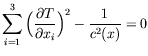(88)

We show how a well established result for this equation can be derived from the wave equation. Then the method of characteristics used to solve this equation is brieflydetailed. The aim of this method is to find a curve called, the characteristic, connecting the source to the receiver and along which the Eikonal equation is transformed into a system of ODEs called a characteristic system. So the unknown travel time can be computed using a simple formula of quadrature. Solving the forward problem using the method of characteristics is done by a specific code named ANRAY. To run a simulation with this code, the density and the wave velocity of each layer have to be specified as well as the position of the source and the receivers. Cubic B-splines are used to interpolate the interfaces and velocity fields.

In our two-dimensional inverse problem model, only one source and eight receivers are considered, so eight shots in total. The interface is defined by the depth of 30 points evenly spaced along the x-axis and the field velocity by a regular mesh. Many domain sizes have been tested with many different forms of interfaces. The inverse problem consists of minimizing the non linear mean square error function: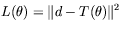(89)

where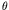is the vector of unkowns (the depth of the nodes of the interface and the value of the velocity at the mesh nodes). Many constraints arise when solving this problem. This function is discontinuous because of the fact that there is no guarantee to find a seismic ray connecting source to receiver for physical or numerical reasons. Moreover this function is very flat about the solution which makes the optimization harder. The simulated annealing algorithm is used to optimize this function. It has the advantage of giving the user the possibility of having only smooth iterations (from the proposed distribution). This can guarantee a unique solution for the problem and which is acceptable physically.

A sensitivity analysis is carried out to determine the maximal acceptable error added to the travel time measurements above which the solution is not reliable. We denote F, the forward problem operator,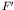its Jacobian matrix, s the vector of singular values ofand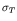the relative error in the travel time measures. We show that in order to have all the parameters of the solution identifiable, the following relationship must hold: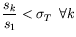(90)

So we can deduce that the threshold of error is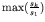.

We show in this paper a method to obtain the velocity field and the shape of the interface of a geological layer from a small number of travel time measures corresponding to a refracted seismic rays. The cost of the computations is affordable and solutions with good accuracy have been obtained. A sensitivity analysis has been made to justify the level of noise added to the measurements.

purchase the full-text of this paper (price £20)